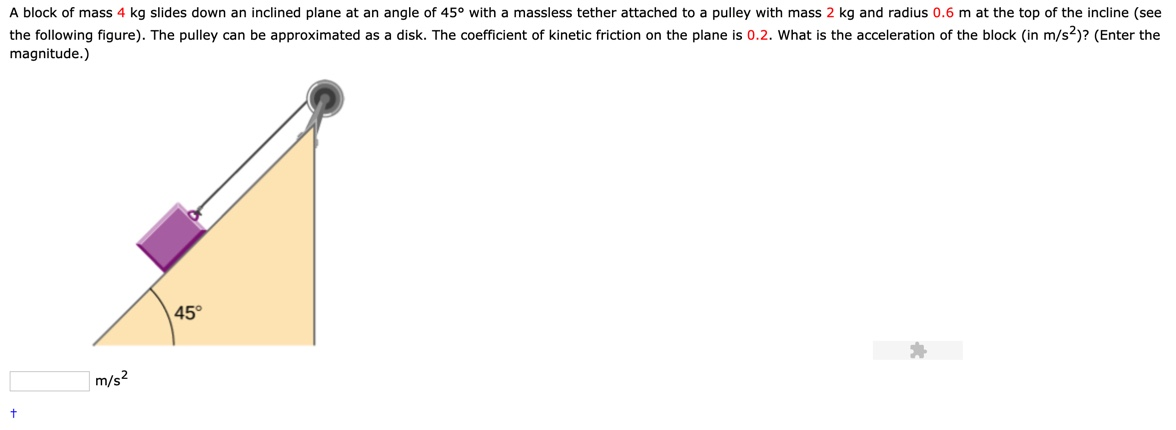# A block of mass 4 kg slides down an inclined plane at an angle of 45° with a massless tether attached to a pulley with mass 2 kg and radius 0.6 m at the top of the incline (see the following figure). The pulley can be approximated as a disk. The coefficient of kinetic friction on the plane is 0.2. What is the acceleration of the block (in m/s2)? (Enter the magnitude.) 45 m/s2 t

Questionhelp_outlineImage TranscriptioncloseA block of mass 4 kg slides down an inclined plane at an angle of 45° with a massless tether attached to a pulley with mass 2 kg and radius 0.6 m at the top of the incline (see the following figure). The pulley can be approximated as a disk. The coefficient of kinetic friction on the plane is 0.2. What is the acceleration of the block (in m/s2)? (Enter the magnitude.) 45 m/s2 t fullscreen

### Want to see the step-by-step answer?